# Summary ray diagrams

At 2F (at two principal focal lengths).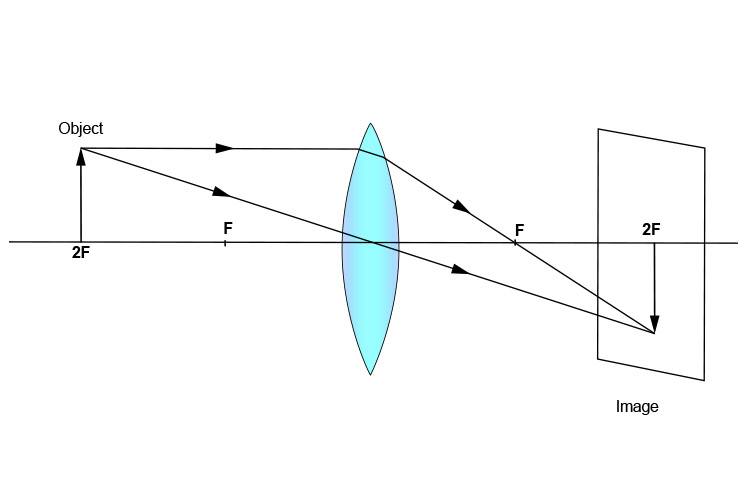At 1F (at one principal focal length).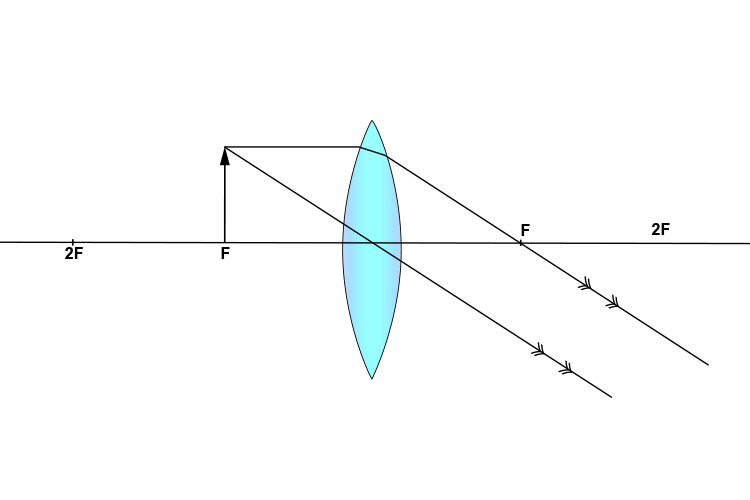At greater than 2F (at more than two principal focal lengths).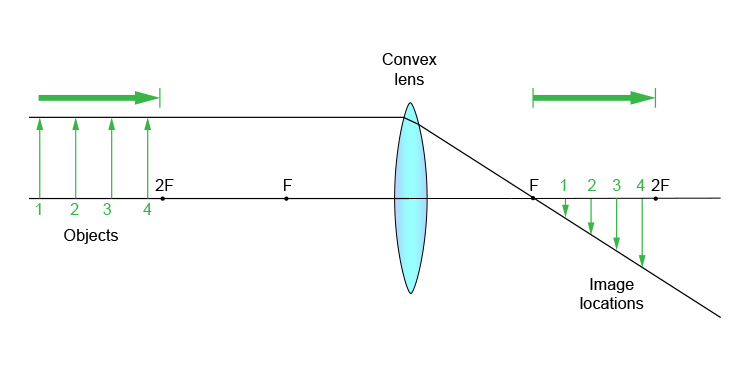Between 2F and F (between two principal focal lengths and one principal focal length).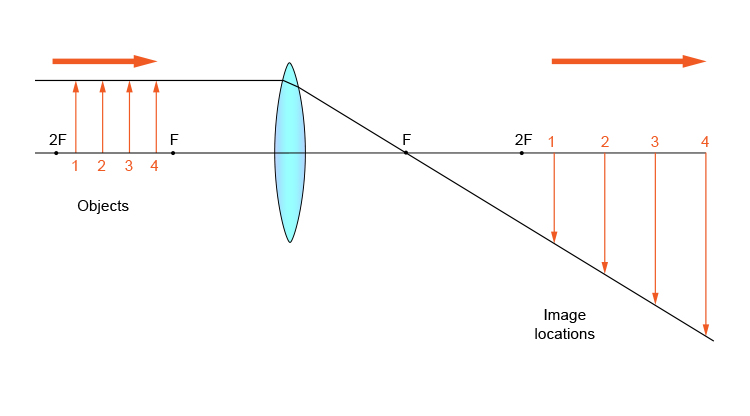Between F and lens (between one principal focal lengths and the lens).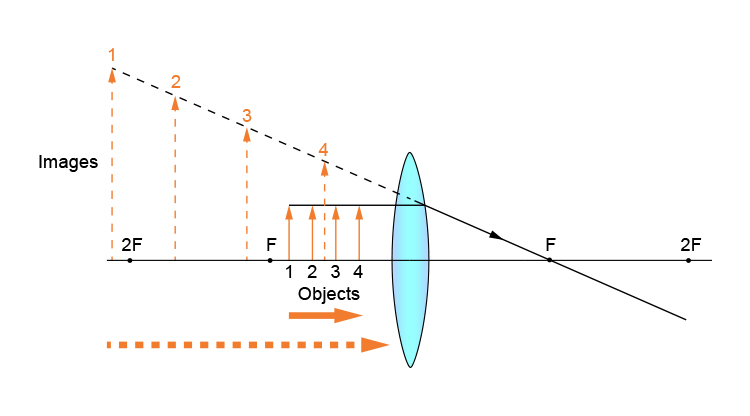and a summary in block format would be.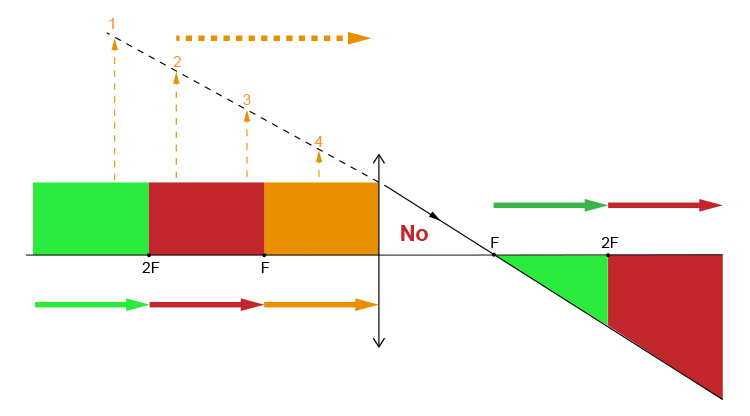NOTE: The NO is to show that there is nothing in this triangle.